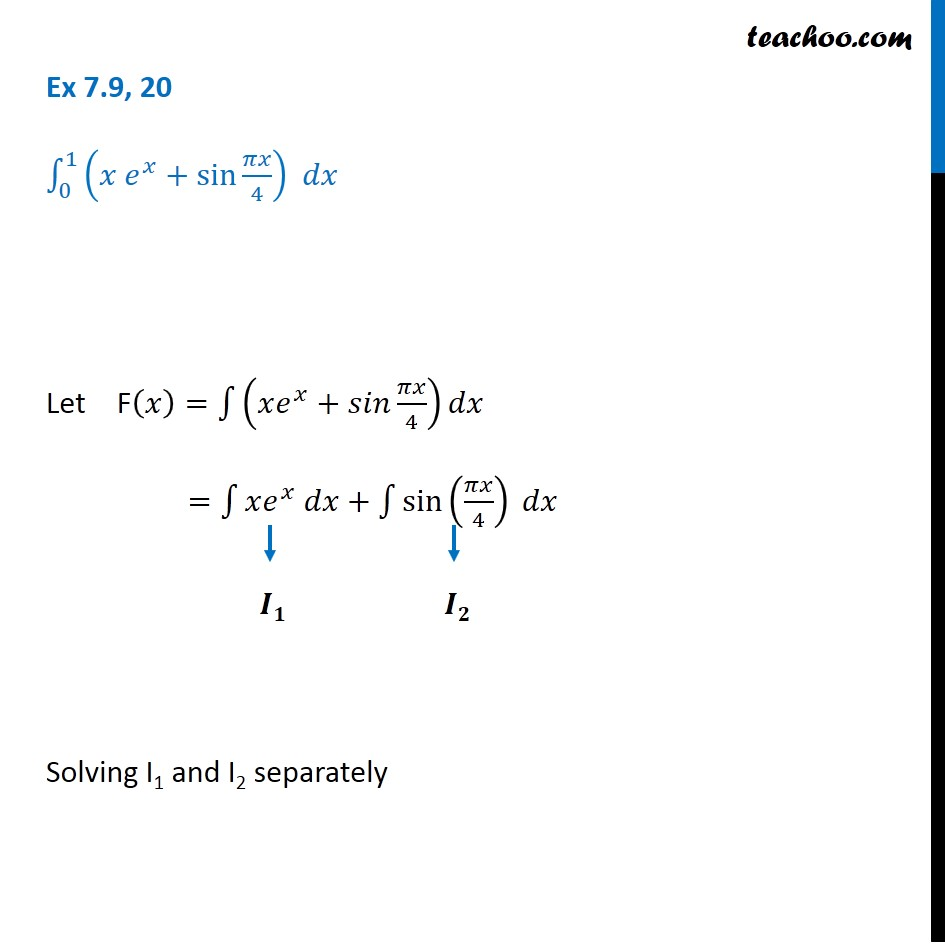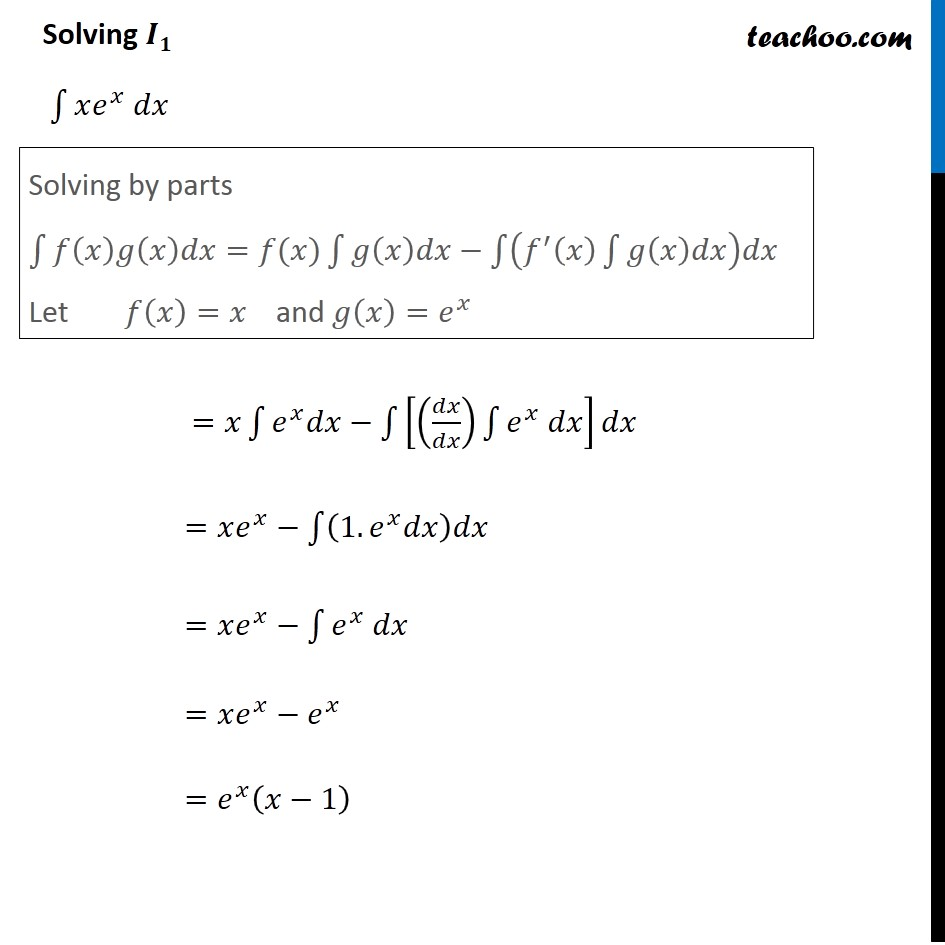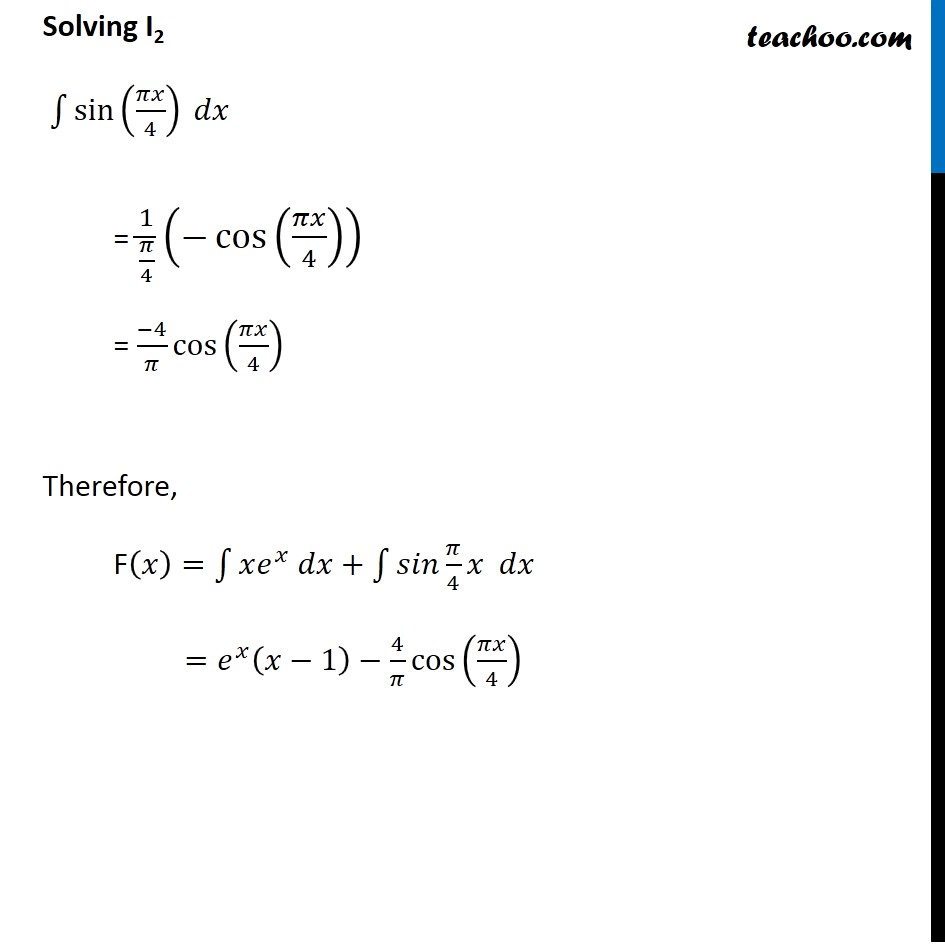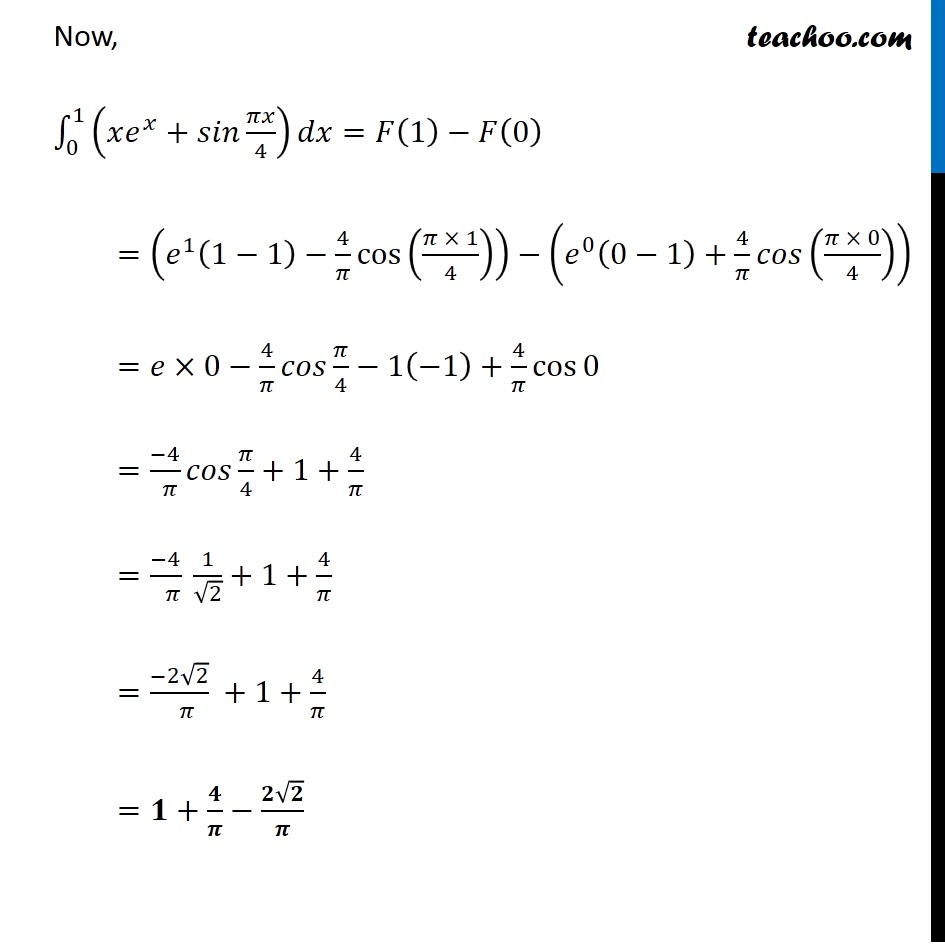Ex 7.9

Chapter 7 Class 12 Integrals
Serial order wiseGet live Maths 1-on-1 Classs - Class 6 to 12

### Transcript

Ex 7.9, 20 ∫_0^1▒(𝑥 𝑒^𝑥+sin⁡〖𝜋𝑥/4〗 ) 𝑑𝑥 Let F(𝑥)=∫1▒(𝑥𝑒^𝑥+𝑠𝑖𝑛 𝜋𝑥/4)𝑑𝑥 =∫1▒〖𝑥𝑒^𝑥 𝑑𝑥+∫1▒〖sin⁡(𝜋𝑥/4) 𝑑𝑥〗〗 Solving I1 and I2 separately Solving 𝑰𝟏 ∫1▒〖𝑥𝑒^𝑥 𝑑𝑥〗 =𝑥∫1▒〖𝑒^𝑥 𝑑𝑥−∫1▒[(𝑑𝑥/𝑑𝑥) ∫1▒〖𝑒^𝑥 𝑑𝑥〗]𝑑𝑥〗 =𝑥𝑒^𝑥−∫1▒(1.𝑒^𝑥 𝑑𝑥)𝑑𝑥 =𝑥𝑒^𝑥−∫1▒〖𝑒^𝑥 𝑑𝑥〗 =𝑥𝑒^𝑥−𝑒^𝑥 =𝑒^𝑥 (𝑥−1) Solving by parts ∫1▒〖𝑓(𝑥)𝑔(𝑥)𝑑𝑥=𝑓(𝑥)∫1▒〖𝑔(𝑥)𝑑𝑥−∫1▒(𝑓^′ (𝑥) ∫1▒𝑔(𝑥)𝑑𝑥)𝑑𝑥〗〗 Let 𝑓(𝑥)=𝑥 and 𝑔(𝑥)=𝑒^𝑥 Solving I2 ∫1▒〖sin⁡(𝜋𝑥/4) 𝑑𝑥〗 = 1/(𝜋/4) (−cos⁡(𝜋𝑥/4) ) = (−4)/𝜋 cos⁡(𝜋𝑥/4) Therefore, F(𝑥)=∫1▒〖𝑥𝑒^𝑥 𝑑𝑥+∫1▒〖𝑠𝑖𝑛 𝜋/4 𝑥 𝑑𝑥〗〗 =𝑒^𝑥 (𝑥−1)−4/𝜋 cos⁡(𝜋𝑥/4) Now, ∫_0^1▒(𝑥𝑒^𝑥+𝑠𝑖𝑛 𝜋𝑥/4) 𝑑𝑥=𝐹(1)−𝐹(0) =(𝑒^1 (1−1)−4/𝜋 cos⁡((𝜋 × 1)/4) )−(𝑒^0 (0−1)+4/𝜋 𝑐𝑜𝑠((𝜋 × 0)/4)) =𝑒×0−4/𝜋 𝑐𝑜𝑠 𝜋/4−1(−1)+4/𝜋 cos⁡0 =(−4)/( 𝜋) 𝑐𝑜𝑠 𝜋/4+1+4/𝜋 =(−4)/( 𝜋) 1/√2+1+4/𝜋 =(−2√2)/( 𝜋) +1+4/𝜋 =𝟏+𝟒/𝝅−(𝟐√𝟐)/𝝅